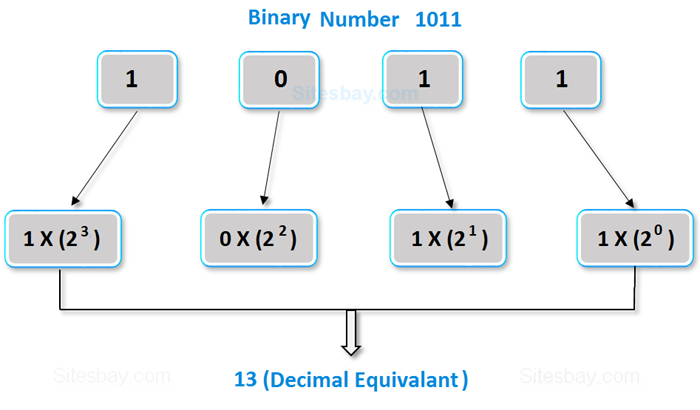# Convert Binary to Decimal in C++

## Program in C++ to Convert Binary to Decimal

In this types of program we takes a binary number as input and converts it into decimal number.• Take a binary number as input.
• Multiply each digits of the binary number starting from the last with the powers of 2 respectively.
• Add all the multiplied digits.
• Here total sum gives the decimal number.

## Convert Binary to Decimal in C

```#include<iostream.h>
#include<conio.h>

void main()
{
clrscr();
long int bin_number, dec_number=0, i=1, rem;
cout<<"Please Enter any Binary Number: ";
cin>>bin_number;
while(bin_number!=0)
{
rem=bin_number%10;
dec_number=dec_number+rem*i;
i=i*2;
bin_number=bin_number/10;
}
cout<<"Equivalent Decimal value:"<<dec_number;
getch();
}
```

## Output

```Please Enter any Binary Number: 1001
Equivalent Decimal value: 9
```

## Output

```Please Enter any Binary Number: 1111
Equivalent Decimal value: 15
```

## Pure VPN Privide Lowest Price VPN Just @ \$1.65. Per Month with Non Detected IP Lowest Price Non Detected IP VPN